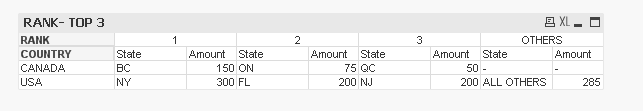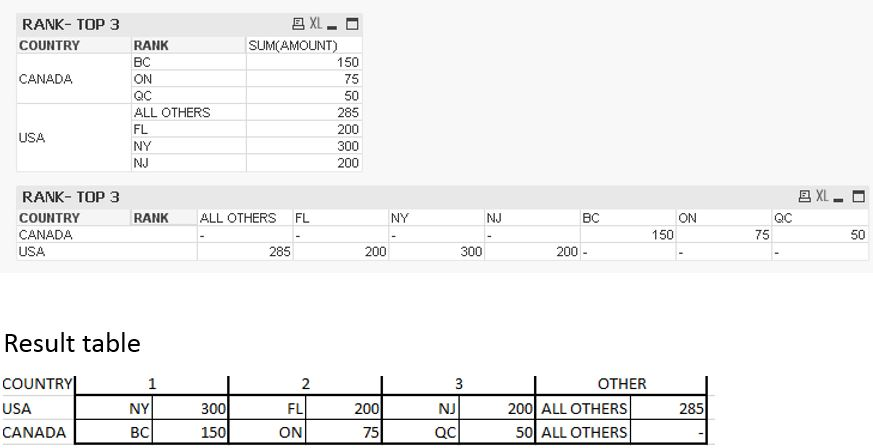# QlikView App Dev

Discussion Board for collaboration related to QlikView App Development.

Announcements
cancel
Showing results for
Did you mean:Creator II

## Rank - List Top values and Group other values

Looking for top 3 states:

1) If there are more than 3 states, all other states besides top 3 should be grouped into 'ALL OTHERS'

2) If there are 3 or less than 3 states, 'All OTHERS' should still be displayed but won't have any value

I used ranked function to create a calculated dimension but how do we create the result table as listed below.

Attached is a qvw with data for you reference. Any experts?

Below is the raw table and result table:

 RAW TABLE COUNTRY STATE AMOUNT USA CA 100 USA TX 125 USA FL 200 USA PA 60 USA NY 300 USA NJ 200 CANADA BC 150 CANADA ON 75 CANADA QC 50

 RESULT TABLE COUNTRY 1 2 3 OTHER USA NY 300 FL 200 NJ 200 ALL OTHERS 285 CANADA BC 150 ON 75 QC 50 ALL OTHERS -
1 Solution

Accepted SolutionsMVP

Here you areDimensions

COUNTRY

=Aggr(If(Rank(Sum(AMOUNT)) < 4, Num(Rank(Sum(AMOUNT), 4)), 'OTHERS'), COUNTRY, STATE)

Expressions

=Aggr(If(Rank(Sum(AMOUNT)) < 4, STATE, 'ALL OTHERS'), COUNTRY, STATE)

SUM(AMOUNT)

6 RepliesMVP

Try this

=Aggr(If(Rank(Sum(AMOUNT)) < 4, STATE, 'ALL OTHERS'), COUNTRY, STATE)Creator II
AuthorThanks Sunny. It's one step forward. I need to produce the final table as the Result table. While pivoting the state, the state is now a column but looking the states for each country to be a row and the corresponding values next to the state.

Any ideas how this can be done?MVP

Here you areDimensions

COUNTRY

=Aggr(If(Rank(Sum(AMOUNT)) < 4, Num(Rank(Sum(AMOUNT), 4)), 'OTHERS'), COUNTRY, STATE)

Expressions

=Aggr(If(Rank(Sum(AMOUNT)) < 4, STATE, 'ALL OTHERS'), COUNTRY, STATE)

SUM(AMOUNT)Creator II
Author

Thanks a lot!!!Creator II
Author

Hi Sunny, in this same example (QVW) if I need to populate 'ALL OTHERS' value for state always how can we achieve that?

'ALL OTHERS' text gets populated if there are more than 3 states. However, If there are 3 or less than 3 states (for instance CANADA that has 3 states) it's blank.MVP

Ya, I guess for non-existent stuff, it might be difficult to populate this...